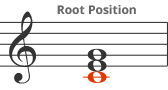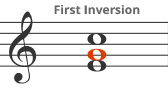« Back to appendix

# Chord Inversions

Any chord can be changed so any other note from the chord can be the lowest note (in terms of sounded pitch). This is called an inversion. In the example below, the C chord is first shown in root position (where the root "C" is the lowest note), then in first inversion (where the third "E" is the lowest note), and finally in second inversion (where the fifth "G" is the lowest note).

root position
Root in Bassfirst inversion
Third in Basssecond inversion
Fifth in Bass